• ## 平衡二叉树

万次阅读 多人点赞 2018-08-15 17:12:47
一、AVL树简介 AVL树的名字来源于它的发明作者G.M....平衡二叉树定义(AVL)：它或者是一颗空树，或者具有以下性质的二叉排序树：它的左子树和右子树的深度之差(平衡因子)的绝对值不超过1，且它的左子树和右子...
一、AVL树简介

AVL树的名字来源于它的发明作者G.M. Adelson-Velsky 和 E.M. Landis。AVL树是最先发明的自平衡二叉查找树（Self-Balancing Binary Search Tree,简称平衡二叉树）。

平衡二叉树定义(AVL)：它或者是一颗空树，或者具有以下性质的二叉排序树：它的左子树和右子树的深度之差(平衡因子)的绝对值不超过1，且它的左子树和右子树都是一颗平衡二叉树。

一棵AVL树有如下必要条件：

条件一：它必须是二叉查找树。
条件二：每个节点的左子树和右子树的高度差至多为1。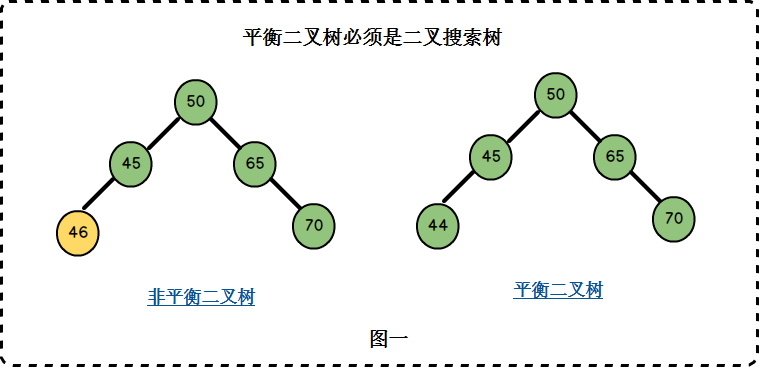图一中左边二叉树的节点45的左孩子46比45大，不满足二叉搜索树的条件，因此它也不是一棵平衡二叉树。
右边二叉树满足二叉搜索树的条件，同时它满足条件二，因此它是一棵平衡二叉树。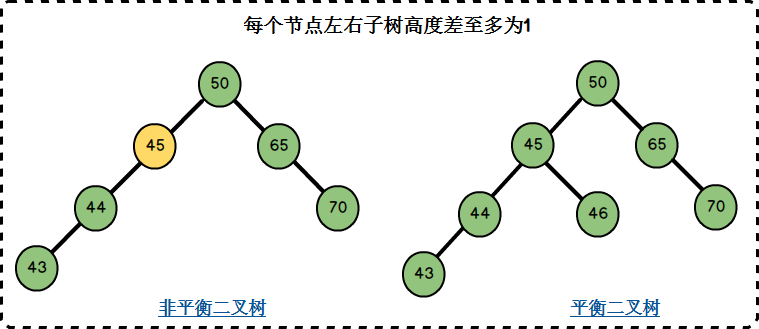左边二叉树的节点45左子树高度2，右子树高度0，左右子树高度差为2-0=2，不满足条件二；
右边二叉树的节点均满足左右子树高度差至多为1，同时它满足二叉搜索树的要求，因此它是一棵平衡二叉树。

AVL树的查找、插入、删除操作在平均和最坏的情况下都是O（logn），这得益于它时刻维护着二叉树的平衡。如果我们需要查找的集合本身没有顺序，在频繁查找的同时也经常的插入和删除，AVL树是不错的选择。不平衡的二叉查找树在查找时的效率是很低的，因此，AVL如何维护二叉树的平衡是我们的学习重点。

二、AVL树相关概念

1. 平衡因子：将二叉树上节点的左子树高度减去右子树高度的值称为该节点的平衡因子BF(Balance Factor)。
在图二右边的AVL树上：
节点50的左子树高度为3，右子树高度为2，BF= 3-2 = 1；
节点45的左子树高度为2，右子树高度为1，BF= 2-1 = 1；
节点46的左子树高度为0，右子树高度为0，BF= 0-0 = 0；
节点65的左子树高度为0，右子树高度为1，BF= 0-1 = -1；
对于平衡二叉树，BF的取值范围为[-1,1]。如果发现某个节点的BF值不在此范围，则需要对树进行调整。

2. 最小不平衡子树：距离插入节点最近的，且平衡因子的绝对值大于1的节点为根的子树.。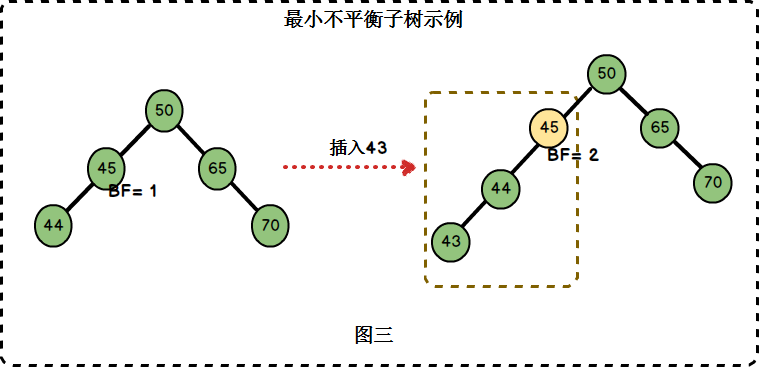在图三中，左边二叉树的节点45的BF = 1，插入节点43后，节点45的BF = 2。节点45是距离插入点43最近的BF不在[-1,1]范围内的节点，因此以节点45为根的子树为最小不平衡子树。

三、AVL树的平衡调整

定义平衡二叉树节点结构：

typedef struct Node
{
int key;
struct Node *left;
struct Node *right;
int height;
}BTNode;

整个实现过程是通过在一棵平衡二叉树中依次插入元素(按照二叉排序树的方式)，若出现不平衡，则要根据新插入的结点与最低不平衡结点的位置关系进行相应的调整。分为LL型、RR型、LR型和RL型4种类型，各调整方法如下(下面用A表示最低不平衡结点)：

1. LL型调整：

由于在A的左孩子(L)的左子树(L)上插入新结点，使原来平衡二叉树变得不平衡，此时A的平衡因子由1增至2。下面图1是LL型的最简单形式。显然，按照大小关系，结点B应作为新的根结点，其余两个节点分别作为左右孩子节点才能平衡，A结点就好像是绕结点B顺时针旋转一样。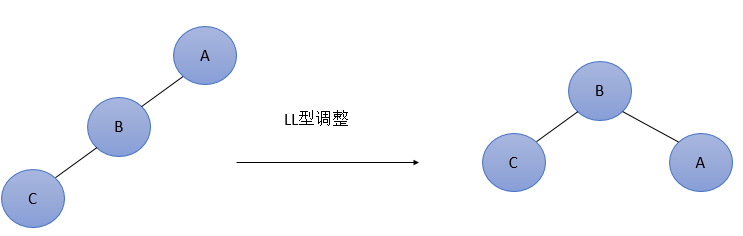LL型调整的一般形式如下图2所示，表示在A的左孩子B的左子树BL(不一定为空)中插入结点(图中阴影部分所示)而导致不平衡( h 表示子树的深度)。这种情况调整如下：①将A的左孩子B提升为新的根结点；②将原来的根结点A降为B的右孩子；③各子树按大小关系连接(BL和AR不变，BR调整为A的左子树)。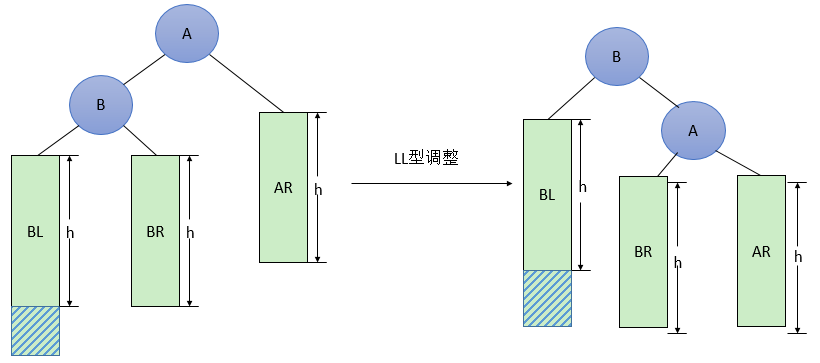图2  一般形式的LL型调整

代码实现：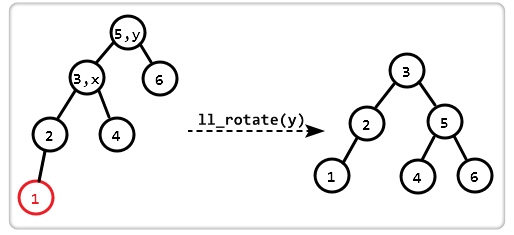BTNode *ll_rotate(BTNode *y)
{
BTNode *x = y->left;
y->left = x->right;
x->right = y;

y->height = max(height(y->left), height(y->right)) + 1;
x->height = max(height(x->left), height(x->right)) + 1;

return x;
}

2. RR型调整：

由于在A的右孩子(R)的右子树(R)上插入新结点，使原来平衡二叉树变得不平衡，此时A的平衡因子由-1变为-2。图3是RR型的最简单形式。显然，按照大小关系，结点B应作为新的根结点，其余两个节点分别作为左右孩子节点才能平衡，A结点就好像是绕结点B逆时针旋转一样。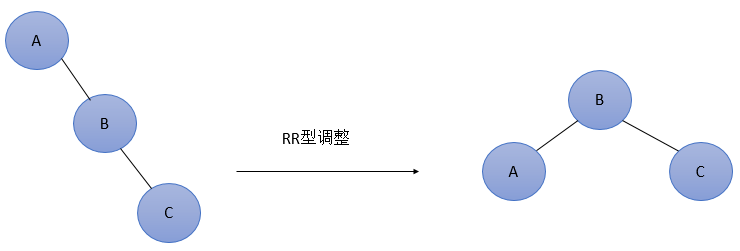RR型调整的一般形式如下图4所示，表示在A的右孩子B的右子树BR(不一定为空)中插入结点(图中阴影部分所示)而导致不平衡( h 表示子树的深度)。这种情况调整如下：

将A的右孩子B提升为新的根结点；
将原来的根结点A降为B的左孩子
各子树按大小关系连接(AL和BR不变，BL调整为A的右子树)。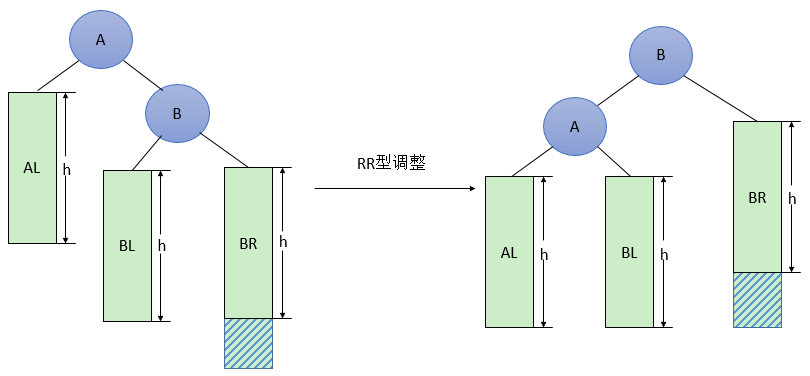图4  一般形式的RR型调整

代码实现：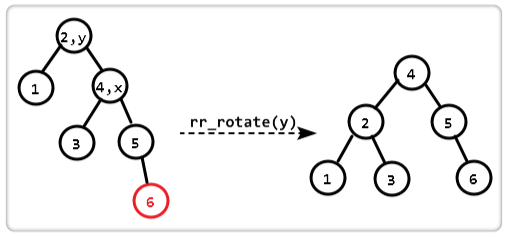BTNode *rr_rotate(struct Node *y)
{
BTNode *x = y->right;
y->right = x->left;
x->left = y;

y->height = max(height(y->left), height(y->right)) + 1;
x->height = max(height(x->left), height(x->right)) + 1;

return x;
}

3. LR型调整

由于在A的左孩子(L)的右子树(R)上插入新结点，使原来平衡二叉树变得不平衡，此时A的平衡因子由1变为2。图5是LR型的最简单形式。显然，按照大小关系，结点C应作为新的根结点，其余两个节点分别作为左右孩子节点才能平衡。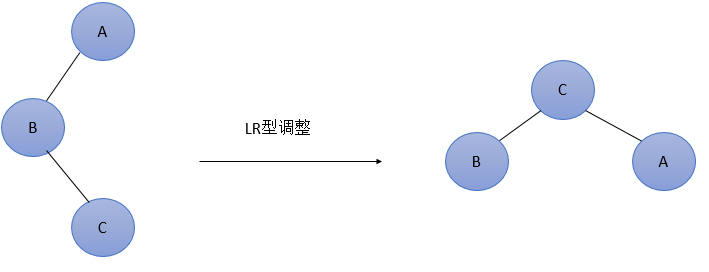图5  最简单的LR型调整

LR型调整的一般形式如下图6所示，表示在A的左孩子B的右子树(根结点为C，不一定为空)中插入结点(图中两个阴影部分之一)而导致不平衡( h 表示子树的深度)。这种情况调整如下：①将B的左孩子C提升为新的根结点；②将原来的根结点A降为C的右孩子；③各子树按大小关系连接(BL和AR不变，CL和CR分别调整为B的右子树和A的左子树)。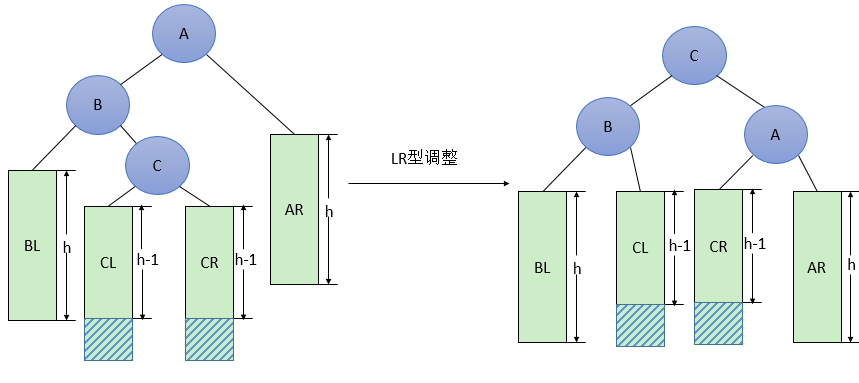图6  一般形式的LR型调整

3.1 代码实现：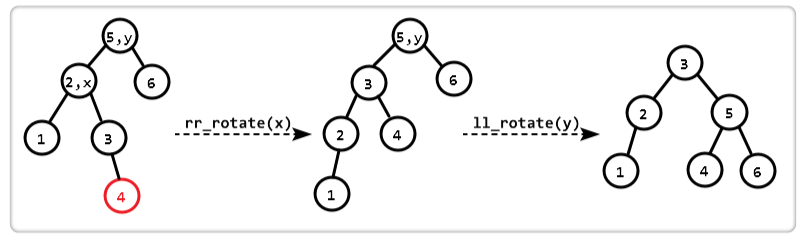BTNode* lr_rotate(BTNode* y)
{
BTNode* x = y->left;
y->left = rr_rotate(x);
return ll_rotate(y);
}

4. RL型调整：

由于在A的右孩子(R)的左子树(L)上插入新结点，使原来平衡二叉树变得不平衡，此时A的平衡因子由-1变为-2。图7是RL型的最简单形式。显然，按照大小关系，结点C应作为新的根结点，其余两个节点分别作为左右孩子节点才能平衡。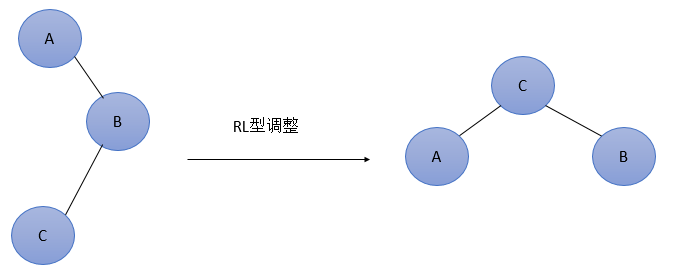图7  最简单的RL型调整

RL型调整的一般形式如下图8所示，表示在A的右孩子B的左子树(根结点为C，不一定为空)中插入结点(图中两个阴影部分之一)而导致不平衡( h 表示子树的深度)。这种情况调整如下：①将B的左孩子C提升为新的根结点；②将原来的根结点A降为C的左孩子；③各子树按大小关系连接(AL和BR不变，CL和CR分别调整为A的右子树和B的左子树)。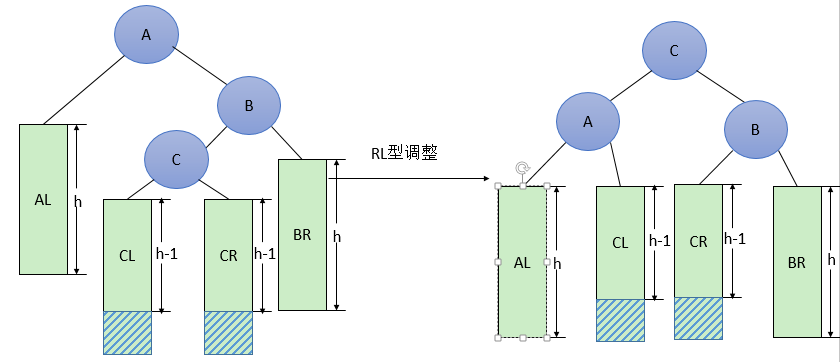图8  一般形式的RL型调整

4.1 代码实现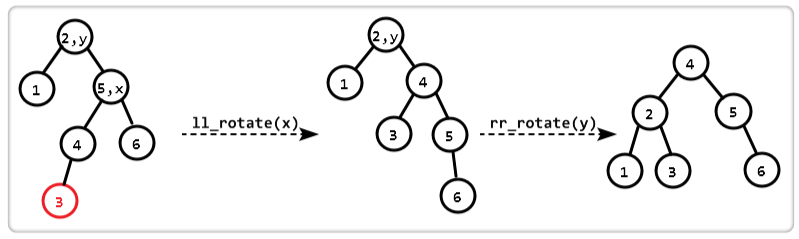Node* rl_rotate(Node* y)
{
Node * x = y->right;
y->right = ll_rotate(x);
return rr_rotate(y);
}

平衡二叉树实现的实例

选取一组数据分别为2，1，0，3，4，5，6，9，8，7的10个结点来构造平衡二叉树。

首先数据为2的结点作为根结点插入，接着插入1，仍是平衡的，再插入0是，2的平衡因子变为2，此时出现了不平衡，因此需要进行调整，最低不平衡结点为2，属于LL型，调整过程如图1所示。

插入操作完整代码

#include<stdio.h>
#include<stdlib.h>

typedef struct Node
{
int key;
struct Node *left;
struct Node *right;
int height;
}BTNode;

int max(int a, int b);

int height(struct Node *N)
{
if (N == NULL)
return 0;
return N->height;
}

int max(int a, int b)
{
return (a > b) ? a : b;
}

BTNode* newNode(int key)
{
struct Node* node = (BTNode*)malloc(sizeof(struct Node));
node->key = key;
node->left = NULL;
node->right = NULL;
node->height = 1;
return(node);
}

BTNode* ll_rotate(BTNode* y)
{
BTNode *x = y->left;
y->left = x->right;
x->right = y;

y->height = max(height(y->left), height(y->right)) + 1;
x->height = max(height(x->left), height(x->right)) + 1;

return x;
}

BTNode* rr_rotate(BTNode* y)
{
BTNode *x = y->right;
y->right = x->left;
x->left = y;

y->height = max(height(y->left), height(y->right)) + 1;
x->height = max(height(x->left), height(x->right)) + 1;

return x;
}

int getBalance(BTNode* N)
{
if (N == NULL)
return 0;
return height(N->left) - height(N->right);
}

BTNode* insert(BTNode* node, int key)
{

if (node == NULL)
return newNode(key);

if (key < node->key)
node->left = insert(node->left, key);
else if (key > node->key)
node->right = insert(node->right, key);
else
return node;

node->height = 1 + max(height(node->left), height(node->right));

int balance = getBalance(node);

if (balance > 1 && key < node->left->key) //LL型
return ll_rotate(node);

if (balance < -1 && key > node->right->key)     //RR型
return rr_rotate(node);

if (balance > 1 && key > node->left->key)     //LR型
{
node->left = rr_rotate(node->left);
return ll_rotate(node);
}

if (balance < -1 && key < node->right->key)     //RL型
{
node->right = ll_rotate(node->right);
return rr_rotate(node);
}

return node;
}

void preOrder(struct Node *root)
{
if (root != NULL)
{
printf("%d ", root->key);
preOrder(root->left);
preOrder(root->right);
}
}

int main()
{
BTNode *root = NULL;

root = insert(root, 2);
root = insert(root, 1);
root = insert(root, 0);
root = insert(root, 3);
root = insert(root, 4);
root = insert(root, 4);
root = insert(root, 5);
root = insert(root, 6);
root = insert(root, 9);
root = insert(root, 8);
root = insert(root, 7);

printf("前序遍历：");
preOrder(root);
return 0;
}

删除代码：

struct Node * minValueNode(BTNode* node)
{
BTNode* current = node;

while (current->left != NULL)
current = current->left;

return current;
}

BTNode* deleteNode(BTNode* root, int key)
{

if (root == NULL)
return root;

if ( key < root->key )
root->left = deleteNode(root->left, key);

else if( key > root->key )
root->right = deleteNode(root->right, key);

else
{
if( (root->left == NULL) || (root->right == NULL) )
{
BTNode* temp = root->left ? root->left :  root->right;

if (temp == NULL)
{
temp = root;
root = NULL;
}
else
*root = *temp;
free(temp);
}
else
{
BTNode* temp = minValueNode(root->right);

root->key = temp->key;

root->right = deleteNode(root->right, temp->key);
}
}

if (root == NULL)
return root;

root->height = 1 + max(height(root->left), height(root->right));

int balance = getBalance(root);

if (balance > 1 && getBalance(root->left) >= 0) //LL型
return ll_rotate(root);

if (balance > 1 && getBalance(root->left) < 0) //LR型
{
root->left =  rr_rotate(root->left);
return ll_rotate(root);
}

if (balance < -1 && getBalance(root->right) <= 0) //RR型
return rr_rotate(root);

if (balance < -1 && getBalance(root->right) > 0)  //Rl型
{
root->right = ll_rotate(root->right);
return rr_rotate(root);
}

return root;
}


完整代码：

#include<stdio.h>
#include<stdlib.h>

typedef struct Node
{
int key;
struct Node *left;
struct Node *right;
int height;
}BTNode;

int max(int a, int b);

int height(struct Node *N)
{
if (N == NULL)
return 0;
return N->height;
}

int max(int a, int b)
{
return (a > b) ? a : b;
}

BTNode* newNode(int key)
{
struct Node* node = (BTNode*)malloc(sizeof(struct Node));
node->key = key;
node->left = NULL;
node->right = NULL;
node->height = 1;
return(node);
}

BTNode* ll_rotate(BTNode* y)
{
BTNode *x = y->left;
y->left = x->right;
x->right = y;

y->height = max(height(y->left), height(y->right)) + 1;
x->height = max(height(x->left), height(x->right)) + 1;

return x;
}

BTNode* rr_rotate(BTNode* y)
{
BTNode *x = y->right;
y->right = x->left;
x->left = y;

y->height = max(height(y->left), height(y->right)) + 1;
x->height = max(height(x->left), height(x->right)) + 1;

return x;
}

int getBalance(BTNode* N)
{
if (N == NULL)
return 0;
return height(N->left) - height(N->right);
}

BTNode* insert(BTNode* node, int key)
{

if (node == NULL)
return newNode(key);

if (key < node->key)
node->left = insert(node->left, key);
else if (key > node->key)
node->right = insert(node->right, key);
else
return node;

node->height = 1 + max(height(node->left), height(node->right));

int balance = getBalance(node);

if (balance > 1 && key < node->left->key) //LL型
return ll_rotate(node);

if (balance < -1 && key > node->right->key)     //RR型
return rr_rotate(node);

if (balance > 1 && key > node->left->key)     //LR型
{
node->left = rr_rotate(node->left);
return ll_rotate(node);
}

if (balance < -1 && key < node->right->key)     //RL型
{
node->right = ll_rotate(node->right);
return rr_rotate(node);
}

return node;
}

BTNode * minValueNode(BTNode* node)
{
BTNode* current = node;

while (current->left != NULL)
current = current->left;

return current;
}

BTNode* deleteNode(BTNode* root, int key)
{

if (root == NULL)
return root;

if (key < root->key)
root->left = deleteNode(root->left, key);

else if (key > root->key)
root->right = deleteNode(root->right, key);

else
{
if ((root->left == NULL) || (root->right == NULL))
{
BTNode* temp = root->left ? root->left : root->right;

if (temp == NULL)
{
temp = root;
root = NULL;
}
else
*root = *temp;
free(temp);
}
else
{
BTNode* temp = minValueNode(root->right);

root->key = temp->key;

root->right = deleteNode(root->right, temp->key);
}
}

if (root == NULL)
return root;

root->height = 1 + max(height(root->left), height(root->right));

int balance = getBalance(root);

if (balance > 1 && getBalance(root->left) >= 0) //LL型
return ll_rotate(root);

if (balance > 1 && getBalance(root->left) < 0) //LR型
{
root->left = rr_rotate(root->left);
return ll_rotate(root);
}

if (balance < -1 && getBalance(root->right) <= 0) //RR型
return rr_rotate(root);

if (balance < -1 && getBalance(root->right) > 0)  //Rl型
{
root->right = ll_rotate(root->right);
return rr_rotate(root);
}

return root;
}

void preOrder(struct Node *root)
{
if (root != NULL)
{
printf("%d ", root->key);
preOrder(root->left);
preOrder(root->right);
}
}

int main()
{
BTNode *root = NULL;

root = insert(root, 9);
root = insert(root, 5);
root = insert(root, 10);
root = insert(root, 0);
root = insert(root, 6);
root = insert(root, 11);
root = insert(root, -1);
root = insert(root, 1);
root = insert(root, 2);
printf("前序遍历：\n");
preOrder(root);

/* The constructed AVL Tree would be
9
/  \
1    10
/  \     \
0    5     11
/    /  \
-1   2    6
*/

root = deleteNode(root, 10);
/* The AVL Tree after deletion of 10
1
/   \
0     9
/     /  \
-1     5     11
/  \
2    6
*/
printf("\n");
printf("前序遍历：\n");
preOrder(root);
return 0;
}

输出结果：参考资料

GeeksforGeeks. AVL Tree | Set 1 (Insertion).
GeeksforGeeks. AVL Tree | Set 2 (Deletion).
平衡二叉树的实现原理
平衡二叉树的实例
AVL 树

展开全文• 平衡二叉树 为了 避免 二叉树退化成链表的极端情况 即使元素很多，二叉树的深度 【节点到子节点的最远距离 】也只有五层 查找 二叉树的任一元素 通过二分法 最多查找五次就确定了。从而 一颗二叉树 查找...
HashMap  在Jdk1.8 以后   在链表长度超过8以后  把连接转换成 红黑树了

原因：  红黑树 效率更高效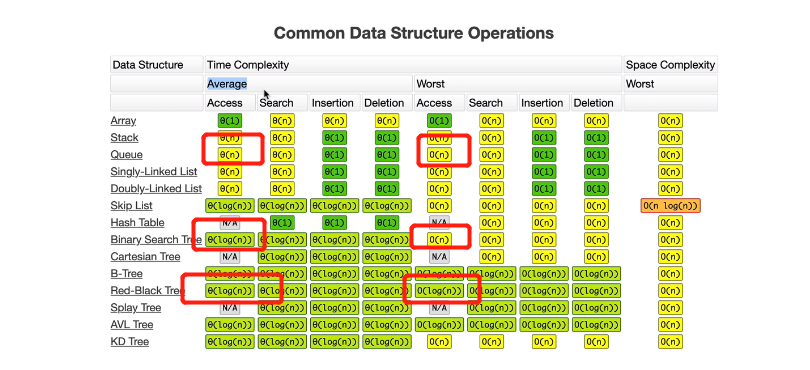特殊情况  ： 二叉树 退化成链表了  左右两端不平衡，那么此时 二叉树的查询时间  和  链表一样都是O(n)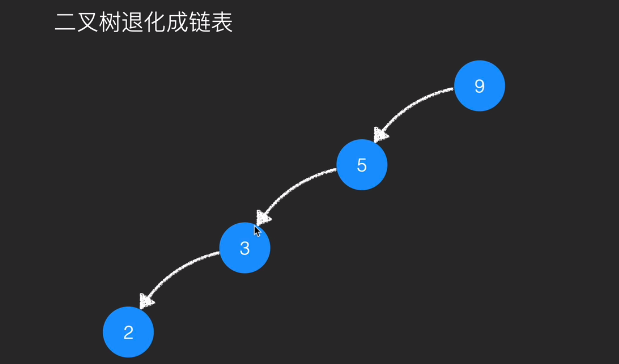一 平衡二叉树  为了 避免 二叉树退化成链表的极端情况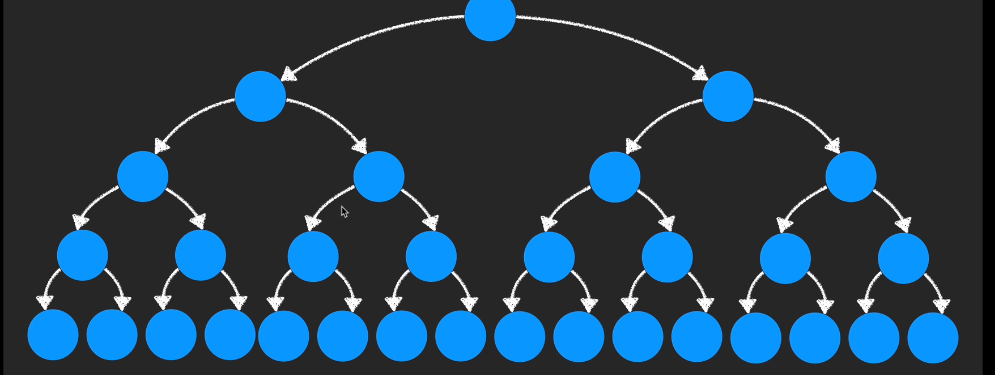即使元素很多，二叉树的深度 【根节点到子节点的最远距离 】也只有五层查找 二叉树的任一元素 通过二分法 最多查找五次就确定了。从而 一颗二叉树 查找效率是和树的高度有关系的。

而平衡二叉树 就是通过某种方式，来保证 一个二叉树 不会退化成链表；

判断平衡被破坏： 通过左右2个子节点的高度差 来看的   差>1  表明不平衡

平衡二叉树的处理方案： 旋转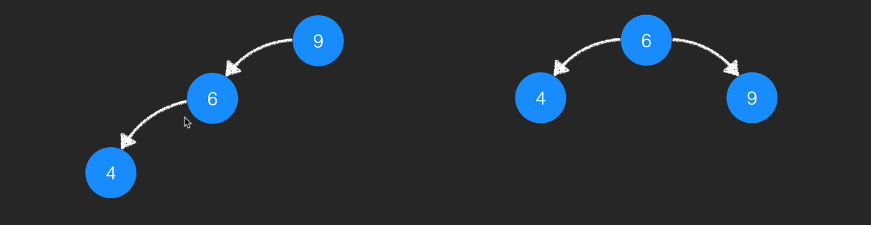多元素处理：

这时是平衡状态  左侧高度是2  右侧是1   高度差是1  平衡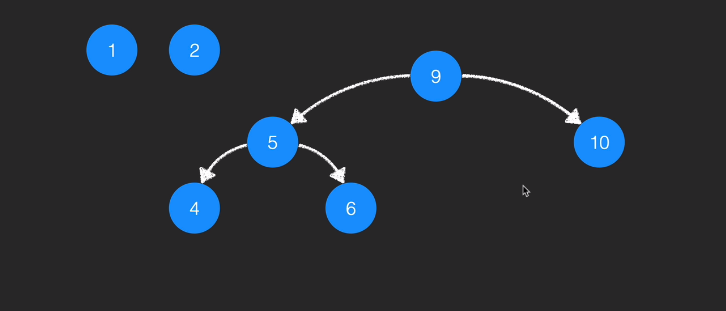如果再添加一个元素的话：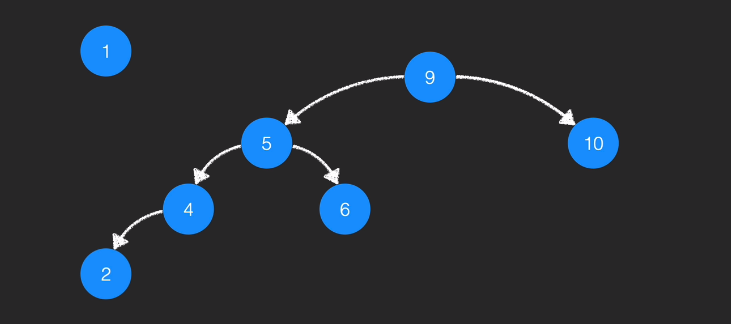操作后：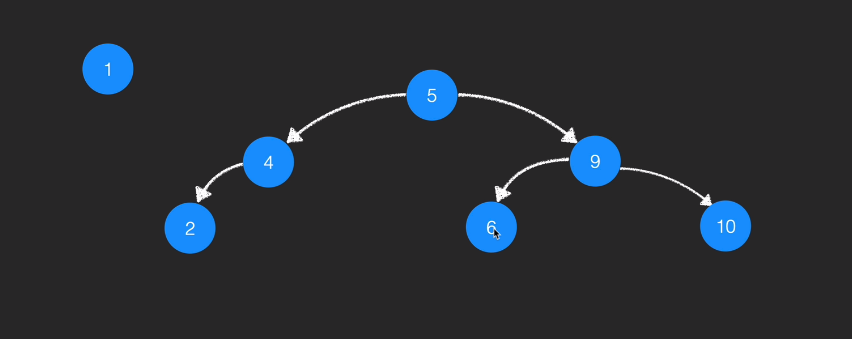二  红黑树 是不是平衡二叉树？

严格意义上 不是平衡二叉树，它不能保证 左右节点高度差不能超过1 ，因为平衡二叉树 获得平衡 很容易触发旋转的操作，，红黑树为了获得比平衡二叉树，更高的插入和删除的效率，在维持树的平衡上 做出了一定的取舍，从而在整体性能【因为查询上不一定优于平衡二叉树】上是优于 平衡二叉树的。


展开全文• 二叉树与平衡二叉树 导语：学过数据结构或者算法的人都应该知道树的概念，简言之树就是由结点或顶点和边组成的(可能是非线性的)且不存在着任何环的一种数据结构；没错在简洁点就是一种数据结构。 然而树又分为好多...
二叉树与平衡二叉树
导语：学过数据结构或者算法的人都应该知道树的概念，简言之树就是由结点或顶点和边组成的(可能是非线性的)且不存在着任何环的一种数据结构；没错在简洁点就是一种数据结构。
然而树又分为好多类，比如：：

无序树：树中任意节点的子结点之间没有顺序关系，这种树称为无序树；
有序树：树中任意节点的子结点之间有顺序关系，这种树称为有序树；
二叉树：每个节点最多含有两个子树的树称为二叉树；
满二叉树：一个二叉树，如果每一个层的结点数都达到最大值
哈夫曼树：带权路径最短的二叉树称为哈夫曼树或最优二叉树；
完全二叉树：当且仅当其每一个结点都与深度为K的满二叉树中编号从1至n的结点一一对应时称之为完全二叉树
平衡二叉树：一棵空树或它的左右两个子树的高度差的绝对值不超过1，并且左右两个子树都是一棵平衡二叉树

… … … …在细分的话还有好多本篇文章主要讲二叉树和平衡二叉树，也是在我们平常面试中面试官比较爱问并且最最最基础的两种~
二叉树：
概念
二叉树是一个连通的无环图，并且每一个顶点的度不大于3。有根二叉树还要满足根结点的度不大于2。有了根结点之后，每个顶点定义了唯一的父结点，和最多2个子结点
性质

二叉树的每个结点至多只有二棵子树(不存在度大于2的结点)，二叉树的子树有左右之分，次序不能颠倒
二叉树的第 i 层至多有 2i−12i−1 个结点
深度为 k 的二叉树至多有 2k−12k−1 个结点
对任何一棵二叉树T，如果其终端结点数为n0，度为2的结点数为n2，则n0=n2+1
一棵深度为k，且有 2k−12k−1 个节点称之为满二叉树
深度为k，有n个节点的二叉树，当且仅当其每一个节点都与深度为k的满二叉树中，序号为1至n的节点对应时，称之为完全二叉树

数据结构
二叉树由一系列树结点组成，每个节点包括三部分：存储数据元素的数据域、存储左子结点地址的指针域、存储右子结点地址的指针域
1.二叉树的代码实现
public class TreeNode{
public int val;//数据域
public TreeNode left；//左子树根结点
public TreeNode right;//右子树根结点
public TreeNode{}
public TreeNode（int val）{this.val = val;}
}
2.二叉树的先序遍历(根结点->左子结点->右子节点)
//递归
public static void preOrder(TreeNode root){
if(root==null){
return ;
}
System.out.println(roor.val+"->");
preOrder(root.left);
preOrder(root.right);
}
//非递归1（通过辅助栈）=====================================
public static void preOrder1(TreeNode root){
if(root==null){
return;
}
Stack<TreeNode> stack = new Stack<>();//创建一个辅助栈
TreeNode cur = root;
while(cur!=null || !stack.isEmpty()){
while(cur!=null){//不断将左子节点入栈，直到cur为空
stack.push(cur);
System.out.println(cur.val+"->");//依次将根结点，左子结点，右子结点加到栈中
cur = cur.left;
}
if(!stack.isEmpty){//栈不为空，弹出栈元素
cur = stack.pop();//弹出最左边的结点
cur = cur.right;//令当前节点为右子结点
}
}
}
//非递归2（通过辅助栈）==================================
public static void preOrder2(TreeNode root){
if(root==null){
return;
}
Stack<TreeNode> stack = new Stack<>();//辅助栈保存树的结点
while(!stack.isEmpty()){
TreeNode temp = stack.pop();
System.out.println(root.val+"->")；//先根结点
if(temp.right!=null){//后进先出原则，所以先添加右子结点
}
if(temp.left!=null){
}
}
}
3.二叉树的中序遍历（左子结点->根结点->右子节点）
//递归
public static void midOrder(TreeNode root){
if(root==null){
return ;
}
midOrder(root.left);
System.out.println(roor.val+"->");
midOrder(root.right);
}
//非递归(通过辅助栈)====================================
//先将根结点的左孩子添加到栈内，之后输出栈顶元素，再处理栈顶元素的右子树
public static void midOrder1(TreeNode root){
if(root==null){
return ;
}
Stack<TreeNode> stack = new Stack<>();//辅助栈
TreeNode cur = root;
while(cur!=null || !stack.isEmpty()){
while(cur!=null){// 不断将左子节点入栈，直到cur为空
stack.push(cur);
cur = cur.left;
}
if(!stack.isEmpty()){// 栈不为空，弹出栈元素
cur = stack.pop();// 此时弹出最左边的节点
System.out.println(root.val+"->")；//先打印左子结点在打印当前结点，然后再把右子节点加到栈中
cur = cur.right;//令当前节点为右子结点
}
}
}
4.二叉树的后序遍历
//递归
public static void postOrder(TreeNode root){
if(root==null){
return ;
}
postOrder(root.left);
postOrder(root.right);
System.out.println(roor.val+"->");
}
//非递归（通过双栈辅助）=================================
public static void postOrder1(TreeNode root){
if(root == null) {
return;
}
Stack<TreeNode> stack1 = new Stack<>();//保存树节点
Stack<TreeNode> stack2 = new Stack<>();//保存（后序）遍历后结果
while(!stack.isEmpty()){
TreeNode temp = stack1.pop();// 将弹出的元素加到stack2中
stack2.push(stack1);
if(temp.left!=null){// 左子结点先入栈
stack1.push(temp.left);
}
if(temp.right!=null){//右子结点后入栈
stack1.push(temp.right)
}
}
while(!stack2.isEmpty()){
System.out.println(stack2.pop().val+"->");//遍历输出
}
}
5.二叉树的层次遍历
//分层遍历二叉树（按层次从上到下，从左到右）迭代，
//原理类似于广度优先搜索，使用队列实现
//队列初始化，将根节点压入队列
//当队列不为空时，弹出一个节点，访问，若左子节点或右子节点不为空，将其压入队列
public static void level(TreeNode root){
if(root == null) {
return;
}
while(!queue.isEmpty()){
TreeNode temp = queue.poll();
System.out.println(temp.val+"->");
if (temp.left != null) { // 添加左右子节点到对列
}
if (temp.right != null) {
}
}
}
6.求二叉树结点的个数
//递归
public static int nodeNumber(TreeNode root){
if(root==null){
return 0;
}
return nodeNumber(root.reft)+nodeNumber(root.right);
}
//非递归法=============================================
public static int nodeNumber1(TreeNode root){
if(root==null){
return 0;
}
int count = 1;// 节点数量
while(!queue.isEmpty()){
TreeNode temp = queue.poll();//每次从对列中删除节点，并返回该节点信息
if(temp.left!=null){// 添加左子结点到对列
count++;
}
if(temp.right!=null){// 添加右子结点到对列
count++;
}
}
return count;
}
7.求二叉树的深度
//递归
//通过递归方法可分别求出左右子树的深度，最后返回深度大的
public int TreeDepth(TreeNode root){
if(root==null){
return 0;
}
int left = TreeDepth(root.left);
int right = TreeDepth(root.right);
return (left>right)?(left+1):(right+1);
//或者可以更简洁的写法如下：
/**return Math.max(getDepthRec(root.left), getDepthRec(root.right)) + 1;*/
}
//非递归

8.求二叉树第K层结点个数
public static int getNodeNum(TreeNode root,k){
if(root==null){
return 0;
}
if(k==1){//只有一个根结点
return 1;
}
//返回root左子树中k-1层的结点个数与root右子树k-1层节结点个数之和
return getNodeNum(root.left,k-1)+getNodeNum(root.right,k-1);
}
9.求二叉树中叶子结点个数
//递归法
public static int getNodeNum(TreeNode root){
if(root==null){//二叉树为空，返回0
return 0;
}
if(root.left==null && root.right==null){//为叶子结点
return 1;
}
//二叉树的叶子节点数 = 左子树叶子节点数 + 右子树叶子节点数
return getNodeNum(root.left)+getNodeNum(root.right);
}
//非递归法（利用Queue进行层次遍历求解）===========================
public static int getNodeNum1(TreeNode root){
if(root==null){
return 0;
}
int leaf = 0;//初始化叶子结点个数
while(!queue.isEmpty()){
TreeNode temp= queue.poll();
if(temp.left==null && temp.right==null){// 叶子节点
leaf++;
}
if(temp.left!=null){
}
if(temp.right!=null){
}
}
return leaf;
}
10判断两棵树是否相同
public static boolean isSame(TreeNode root1,TreeNode root2){
if(root1==null && root2==null){//都为空，返回真
return true;
}else if(root1==null || root2==null){//一个为空，一个不为空
return false;
}
if(root1.val != root2.val){// 两树不为空，但是值不相同
return false;
}
// 递归遍历左右子节点
return isSameRec(r1.left, r2.left) && isSameRec(r1.right, r2.right);
}
平衡二叉树：
概念及性质
平衡二叉树又被称为AVL树（区别于AVL算法），它是一棵二叉排序树，且具有以下性质：它是一棵空树或它的左右两个子树的高度差的绝对值不超过1，并且左右两个子树都是一棵平衡二叉树
数据结构
说到平衡二叉树的数据结构，其实和树是一样的，毕竟平衡二叉树是一种特殊的树，所以他们基本一样。但为什么要单独说一下平衡二叉树呢？因为平衡二叉树最基本、也是最常见的一种算法就是：判断该树是否为平衡二叉树，这里我们还是以代码的方式来介绍：
判断该树是否为平衡二叉树的步骤：

求树的深度
判断是否为平衡树

public class TreeNode{
//判断是否为平衡二叉树
public Boolean isBalance(){
if(root==null){
return true;  //为空肯定是平衡二叉树了
}
int left = TreeDepth(root.left);
int right = TreeDepth(root.right);
int diff = left - right;
if(diff>1||diff<-1){  //左右子树绝对值差大于1，不是平衡二叉树
return false;
}
return isBalance(root.left) && isBalance(root.right); 递归判断左右子树

}
//求二叉树的深度
public int TreeDepth(TreeNode root){
if(root==null){
return 0;
}
int left = TreeDepth(root.left);
int right = TreeDepth(root.right);
return (left>right)?(left+1):(right+1);
}
}
结语：对于二叉树和平衡二叉树的介绍基本上就到这了，如果有不懂或者对本篇文章有错误疑问的欢迎提问与指正，懂的留个小爪吧哈哈哈


展开全文数据结构
• 平衡二叉树，又称为 AVL 树。实际上就是遵循以下两个特点的二叉树： 每棵子树中的左子树和右子树的深度差不能超过 1； 二叉树中每棵子树都要求是平衡二叉树； 其实就是在二叉树的基础上，若树中每棵子树都满足其左...
平衡二叉树，又称为 AVL 树。实际上就是遵循以下两个特点的二叉树：

每棵子树中的左子树和右子树的深度差不能超过 1；
二叉树中每棵子树都要求是平衡二叉树；
平衡二叉树不唯一，ASL相同即可。
其实就是在二叉树的基础上，若树中每棵子树都满足其左子树和右子树的深度差都不超过 1，则这棵二叉树就是平衡二叉树。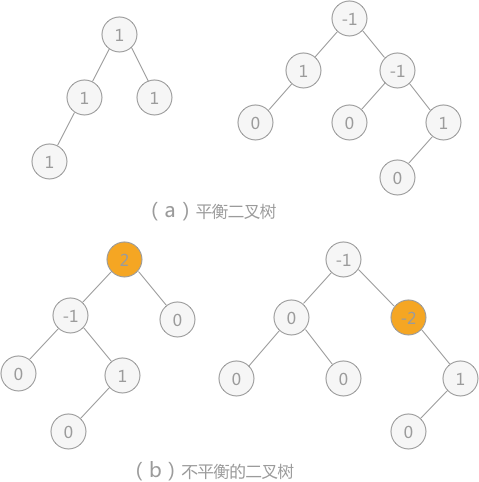平衡因子：每个结点都有其各自的平衡因子，表示的就是其左子树深度同右子树深度的差。平衡二叉树中各结点平衡因子的取值只可能是：0、1 和 -1。
如图 1 所示，其中 （a） 的两棵二叉树中由于各个结点的平衡因子数的绝对值都不超过 1，所以 （a） 中两棵二叉树都是平衡二叉树；而 （b） 的两棵二叉树中有结点的平衡因子数的绝对值超过 1，所以都不是平衡二叉树。
二叉排序树转化为平衡二叉树
为了排除动态查找表中不同的数据排列方式对算法性能的影响，需要考虑在不会破坏二叉排序树本身结构的前提下，将二叉排序树转化为平衡二叉树。
例如，使用上一节的算法在对查找表{13，24，37，90，53}构建二叉排序树时，当插入 13 和 24 时，二叉排序树此时还是平衡二叉树：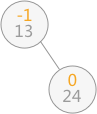当继续插入 37 时，生成的二叉排序树如图 3（a），平衡二叉树的结构被破坏，此时只需要对二叉排序树做“旋转”操作（如图 3（b）），即整棵树以结点 24 为根结点，二叉排序树的结构没有破坏，同时将该树转化为了平衡二叉树：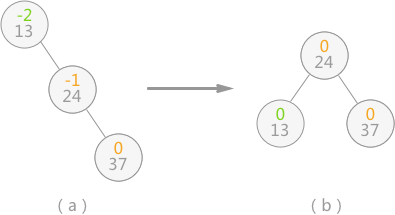当二叉排序树的平衡性被打破时，就如同扁担的两头出现了一头重一头轻的现象，如图3（a）所示，此时只需要改变扁担的支撑点（树的树根），就能使其重新归为平衡。实际上图 3 中的 （b） 是对（a） 的二叉树做了一个向左逆时针旋转的操作。
继续插入 90 和 53 后，二叉排序树如图 4（a）所示，导致二叉树中结点 24 和 37 的平衡因子的绝对值大于 1 ，整棵树的平衡被打破。此时，需要做两步操作：

如图 4（b） 所示，将结点 53 和 90 整体向右顺时针旋转，使本该以 90 为根结点的子树改为以结点 53 为根结点；
如图 4（c） 所示，将以结点 37 为根结点的子树向左逆时针旋转，使本该以 37 为根结点的子树，改为以结点 53 为根结点；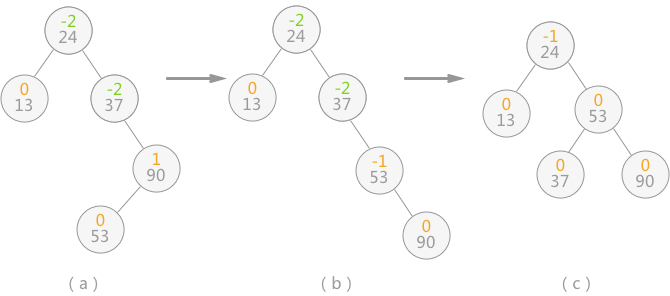做完以上操作，即完成了由不平衡的二叉排序树转变为平衡二叉树。
当平衡二叉树由于新增数据元素导致整棵树的平衡遭到破坏时，就需要根据实际情况做出适当的调整，假设距离插入结点最近的“不平衡因子”为 a。则调整的规律可归纳为以下 4 种情况：

单向右旋平衡处理：若由于结点 a 的左子树为根结点的左子树上插入结点，导致结点 a 的平衡因子由 1 增至 2，致使以 a 为根结点的子树失去平衡，则只需进行一次向右的顺时针旋转，如下图这种情况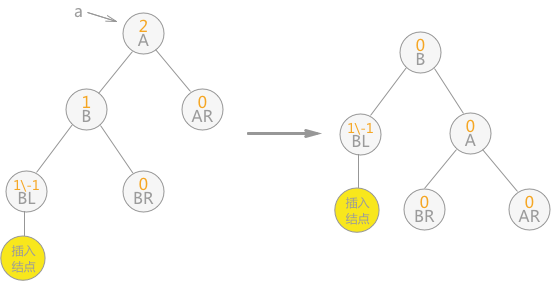单向左旋平衡处理：如果由于结点 a 的右子树为根结点的右子树上插入结点，导致结点 a 的平衡因子由 -1变为 -2，则以 a 为根结点的子树需要进行一次向左的逆时针旋转，如下图这种情况：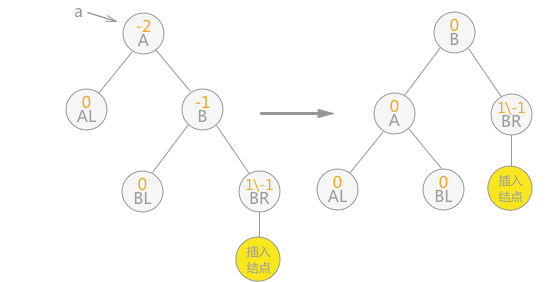双向旋转（先右后左）平衡处理：如果由于结点 a 的右子树为根结点的左子树上插入结点，导致结点 a 平衡因子由 -1 变为 -2，致使以 a 为根结点的子树失去平衡，则需要进行两次旋转（先右旋后左旋）操作，如下图这种情况：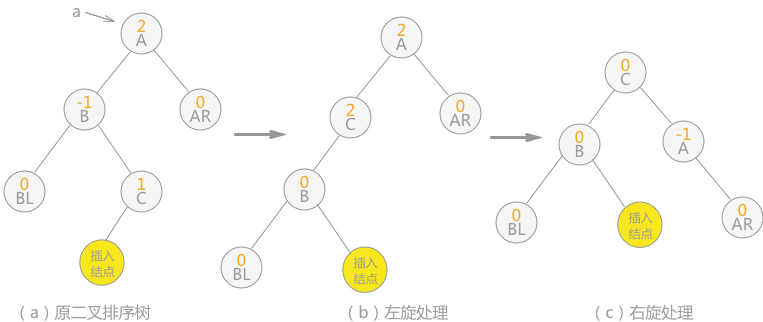注意：图 7 中插入结点也可以为结点 C 的右孩子，则（b）中插入结点的位置还是结点 C 右孩子，（c）中插入结点的位置为结点 A 的左孩子。

双向旋转（先右后左）平衡处理：如果由于结点 a 的右子树为根结点的左子树上插入结点，导致结点 a 平衡因子由 -1 变为 -2，致使以 a 为根结点的子树失去平衡，则需要进行两次旋转（先右旋后左旋）操作，如下图这种情况：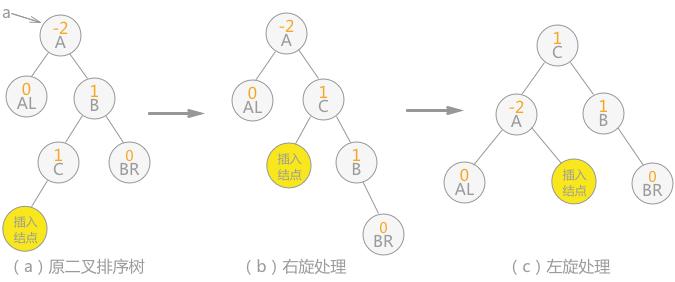注意：图 8 中插入结点也可以为结点 C 的右孩子，则（b）中插入结点的位置改为结点 B 的左孩子，（c）中插入结点的位置为结点 B 的左孩子。

在对查找表{13，24，37，90，53}构建平衡二叉树时，由于符合第 4 条的规律，所以进行先右旋后左旋的处理，最终由不平衡的二叉排序树转变为平衡二叉树。
构建平衡二叉树的代码实现

#include <stdio.h>
#include <stdlib.h>
//分别定义平衡因子数
#define LH +1
#define EH  0
#define RH -1
typedef int ElemType;
typedef enum {false,true} bool;
//定义二叉排序树
typedef struct BSTNode{
ElemType data;
int bf;//balance flag
struct BSTNode *lchild,*rchild;
}*BSTree,BSTNode;
//对以 p 为根结点的二叉树做右旋处理，令 p 指针指向新的树根结点
void R_Rotate(BSTree* p)
{
//借助文章中的图 5 所示加以理解，其中结点 A 为 p 指针指向的根结点
BSTree lc = (*p)->lchild;
(*p)->lchild = lc->rchild;
lc->rchild = *p;
*p = lc;
}
对以 p 为根结点的二叉树做左旋处理，令 p 指针指向新的树根结点
void L_Rotate(BSTree* p)
{
//借助文章中的图 6 所示加以理解，其中结点 A 为 p 指针指向的根结点
BSTree rc = (*p)->rchild;
(*p)->rchild = rc->lchild;
rc->lchild = *p;
*p = rc;
}
//对以指针 T 所指向结点为根结点的二叉树作左子树的平衡处理，令指针 T 指向新的根结点
void LeftBalance(BSTree* T)
{
BSTree lc,rd;
lc = (*T)->lchild;
//查看以 T 的左子树为根结点的子树，失去平衡的原因，如果 bf 值为 1 ，则说明添加在左子树为根结点的左子树中，需要对其进行右旋处理；反之，如果 bf 值为 -1，说明添加在以左子树为根结点的右子树中，需要进行双向先左旋后右旋的处理
switch (lc->bf)
{
case LH:
(*T)->bf = lc->bf = EH;
R_Rotate(T);
break;
case RH:
rd = lc->rchild;
switch(rd->bf)
{
case LH:
(*T)->bf = RH;
lc->bf = EH;
break;
case EH:
(*T)->bf = lc->bf = EH;
break;
case RH:
(*T)->bf = EH;
lc->bf = LH;
break;
}
rd->bf = EH;
L_Rotate(&(*T)->lchild);
R_Rotate(T);
break;
}
}
//右子树的平衡处理同左子树的平衡处理完全类似
void RightBalance(BSTree* T)
{
BSTree lc,rd;
lc= (*T)->rchild;
switch (lc->bf)
{
case RH:
(*T)->bf = lc->bf = EH;
L_Rotate(T);
break;
case LH:
rd = lc->lchild;
switch(rd->bf)
{
case LH:
(*T)->bf = EH;
lc->bf = RH;
break;
case EH:
(*T)->bf = lc->bf = EH;
break;
case RH:
(*T)->bf = EH;
lc->bf = LH;
break;
}
rd->bf = EH;
R_Rotate(&(*T)->rchild);
L_Rotate(T);
break;
}
}
int InsertAVL(BSTree* T,ElemType e,bool* taller)
{
//如果本身为空树，则直接添加 e 为根结点
if ((*T)==NULL)
{
(*T)=(BSTree)malloc(sizeof(BSTNode));
(*T)->bf = EH;
(*T)->data = e;
(*T)->lchild = NULL;
(*T)->rchild = NULL;
*taller=true;
}
//如果二叉排序树中已经存在 e ，则不做任何处理
else if (e == (*T)->data)
{
*taller = false;
return 0;
}
//如果 e 小于结点 T 的数据域，则插入到 T 的左子树中
else if (e < (*T)->data)
{
//如果插入过程，不会影响树本身的平衡，则直接结束
if(!InsertAVL(&(*T)->lchild,e,taller))
return 0;
//判断插入过程是否会导致整棵树的深度 +1
if(*taller)
{
//判断根结点 T 的平衡因子是多少，由于是在其左子树添加新结点的过程中导致失去平衡，所以当 T 结点的平衡因子本身为 1 时，需要进行左子树的平衡处理，否则更新树中各结点的平衡因子数
switch ((*T)->bf)
{
case LH:
LeftBalance(T);
*taller = false;
break;
case  EH:
(*T)->bf = LH;
*taller = true;
break;
case RH:
(*T)->bf = EH;
*taller = false;
break;
}
}
}
//同样，当 e>T->data 时，需要插入到以 T 为根结点的树的右子树中，同样需要做和以上同样的操作
else
{
if(!InsertAVL(&(*T)->rchild,e,taller))
return 0;
if (*taller)
{
switch ((*T)->bf)
{
case LH:
(*T)->bf = EH;
*taller = false;
break;
case EH:
(*T)->bf = RH;
*taller = true;
break;
case  RH:
RightBalance(T);
*taller = false;
break;
}
}
}
return 1;
}
//判断现有平衡二叉树中是否已经具有数据域为 e 的结点
bool FindNode(BSTree root,ElemType e,BSTree* pos)
{
BSTree pt = root;
(*pos) = NULL;
while(pt)
{
if (pt->data == e)
{
//找到节点，pos指向该节点并返回true
(*pos) = pt;
return true;
}
else if (pt->data>e)
{
pt = pt->lchild;
}
else
pt = pt->rchild;
}
return false;
}
//中序遍历平衡二叉树
void InorderTra(BSTree root)
{
if(root->lchild)
InorderTra(root->lchild);

printf("%d ",root->data);

if(root->rchild)
InorderTra(root->rchild);
}
int main()
{
int i,nArr[] = {1,23,45,34,98,9,4,35,23};
BSTree root=NULL,pos;
bool taller;
//用 nArr查找表构建平衡二叉树（不断插入数据的过程）
for (i=0;i<9;i++)
{
InsertAVL(&root,nArr[i],&taller);
}
//中序遍历输出
InorderTra(root);
//判断平衡二叉树中是否含有数据域为 103 的数据
if(FindNode(root,103,&pos))
printf("\n%d\n",pos->data);
else
printf("\nNot find this Node\n");
return 0;
}

//运行结果
1 4 9 23 34 35 45 98
Not find this Node

总结
使用平衡二叉树进行查找操作的时间复杂度为 O(logn)。


展开全文数据结构
• ## 平衡二叉树总结

千次阅读 2018-04-20 20:27:11
平衡二叉树相关概念以及性质 平衡二叉树的类结构 LL型失衡以及解决办法 RR型失衡以及解决办法 LR型失衡以及解决办法 RL型失衡以及解决办法 balance和checkBalance函数 完整源码测试 说明 在学二叉平衡树...
• 数据结构的平衡二叉树，自己的思路整理，有图示和部分的代码二叉排序树 数据结构
• 一、平衡二叉树概述 1.1 什么是平衡二叉树 平衡二叉树也叫 AVL 树。平衡二叉树是具有以下特点的二叉查找树：它是一棵空树或它的左右两个子树的高度差的绝对值不超过 1， 并且左右两个子树都是一棵平衡二叉树。 ...数据结构 java 二叉排序树
• ## 平衡二叉树旋转详解

千次阅读 多人点赞 2017-04-29 16:28:56
平衡二叉树的定义（AVL）定义 平衡二叉树或者是一棵空树，或者满足以下的性质：它的左子树和右子树的高度之差的绝对值不超过1，并且左子树和右子树也是一个平衡二叉树。 平衡因子 左子树高度减去右子树的高度的值...二叉搜索树 查找
• 数据结构实验之查找二：平衡二叉树 Time Limit: 400 ms Memory Limit: 65536 KiB Problem Description 根据给定的输入序列建立一棵平衡二叉树，求出建立的平衡二叉树的树根。 Input 输入一组测试数据。......